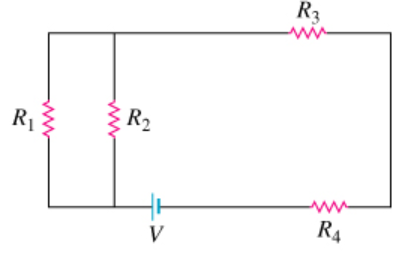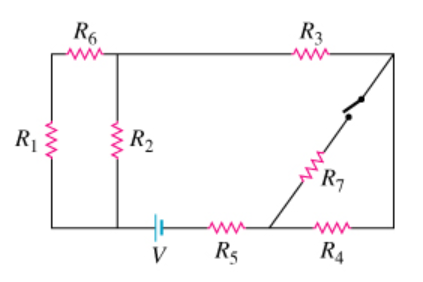# Problem: (a) Consider the network of four resistors shown in the diagram, where R1 = 2.00 Ω, R2 = 5.00 Ω, R3 = 1.00 Ω, and R4 = 7.00 Ω. The resistors are connected to a constant voltage of magnitude V. (Figure 1) Find the equivalent resistance RA of the resistor network.(b) Two resistors of resistance R5 = 3.00 Ω and R6 = 3.00 Ω are added to the network, and an additional resistor of resistance R7 = 3.00 Ω is connected by a switch, as shown in the diagram..(Figure 2) Find the equivalent resistance RB of the new resistor network when the switch is open.(c) Find the equivalent resistance RC of the resistor network described in Part B when the switch is closed.

###### FREE Expert Solution

Equivalent resistance for 2 resistors in parallel:

$\overline{){{\mathbf{R}}}_{{\mathbf{eq}}}{\mathbf{=}}\frac{{\mathbf{R}}_{\mathbf{1}}{\mathbf{R}}_{\mathbf{2}}}{{\mathbf{R}}_{\mathbf{1}}\mathbf{+}{\mathbf{R}}_{\mathbf{2}}}}$

Equivalent resistance for resistors in series:

$\overline{){{\mathbf{R}}}_{{\mathbf{eq}}}{\mathbf{=}}{{\mathbf{R}}}_{{\mathbf{1}}}{\mathbf{+}}{{\mathbf{R}}}_{{\mathbf{2}}}{\mathbf{+}}{\mathbf{.}}{\mathbf{.}}{\mathbf{.}}{\mathbf{+}}{{\mathbf{R}}}_{{\mathbf{n}}}}$

(a)

R1 and R2 are in parallel.

R12 = (2.00)(5.00)/(2.00+5.00) = 1.43Ω

89% (385 ratings)###### Problem Details

(a) Consider the network of four resistors shown in the diagram, where R1 = 2.00 Ω, R2 = 5.00 Ω, R3 = 1.00 Ω, and R4 = 7.00 Ω. The resistors are connected to a constant voltage of magnitude V. (Figure 1) Find the equivalent resistance RA of the resistor network.(b) Two resistors of resistance R5 = 3.00 Ω and R6 = 3.00 Ω are added to the network, and an additional resistor of resistance R7 = 3.00 Ω is connected by a switch, as shown in the diagram..(Figure 2) Find the equivalent resistance RB of the new resistor network when the switch is open.(c) Find the equivalent resistance RC of the resistor network described in Part B when the switch is closed.